C Program to Check Reverse equal Original

In this tutorial you will learn about the C Program to Check Reverse equal Original and its application with practical example.

C Program to Reverse equal Original

In this tutorial, we will learn to create a C program that will Reverse equal Original in C programming.

Prerequisites

Before starting with this tutorial we assume that you are best aware of the following C programming topics:

• Operators in C Programming.
• Basic Input and Output function in C Programming.
• Basic C programming.
• While loop in C programming.

Program to Reverse equal Original:-

As we all know the c is a very powerful language. With the help of c programming language, we can make many programs. We can perform many input-output operations using c programming. In today’s tutorial, we take the input numbers from the user. Then we will Generate Armstrong Numbers between the taken range with the help of the c programming language.

With the help of this program, we can Reverse equal Original.

Output:-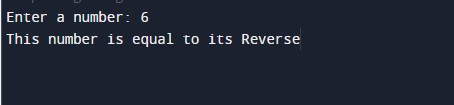In the above program, we have first initialized the required variable.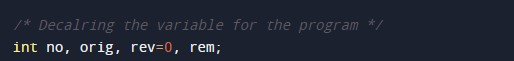• no = it will hold the integer value.
• orig = it will hold the integer value.
• rev = it will hold the integer value.
• rem = it will hold the integer value.

Taking the input integer number from the user.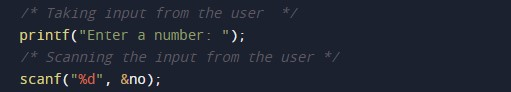Calculating the reverse equal.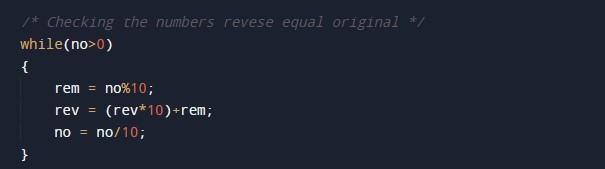Printing the output numbers.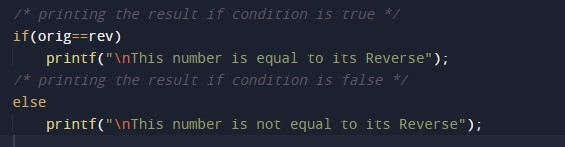In this tutorial we have learn about the C Program to Check Reverse equal Original and its application with practical example. I hope you will like this tutorial.